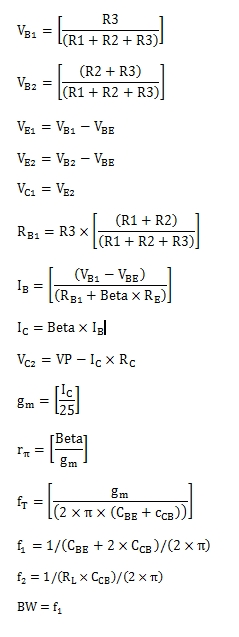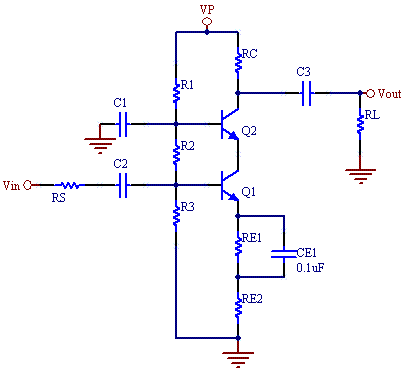# BJT Cascode Amplifier Calculator

This calculator calculates the bias voltage, current, gain and frequency response of a Cascode Amplifier. A cascode amplifier is a two-stage amplifier composed of a transconductance amplifier followed by a current buffer. It can be designed by combining a common-emitter amplifier with a common-base amplifier. This type of amplifier has high gain and high band width, as the Miller capacitance limitations of the common emitter amplifier are minimized by using a second transistor as common- base current buffer.

For this cascode the gain is set by the ratio of RC/RE2.  To increase bandwidth maximize the sum of RE1 and RE2.

Kohms
Kohms
Kohms
Kohms
Kohms
ohms
V
V
ohms
ohms
MHz
pF
pF

#### Result

V
V
V
V
V
mA
mA
V
ohms
Kohms
MHz
MHz
MHz